RUSSIAN JOURNAL OF EARTH SCIENCES, VOL. 16, ES1004, doi:10.2205/2016ES000564, 2016

# Analysis of ionospheric parameters above active seismic regions

Vivek Patil, Roshni Atulkar, P. K. Purohit

National Institute of Technical Teachers' Training and Research, Bhopal, MP, India

### Abstract

 The aim of this paper is to examine the anomalous variations of the critical frequency ($f_oF2$), total electron content (TEC), and $f_oE_s$ parameters before earthquake occurred on 1 April 2014 near Iquique Chile ($M=8.2$) and event on 25 October 2013 in Japan ($M=7.1$). Using the bound method we analyzed the variations of $f_oF2$, TEC, $f_oE_s$ and $N_mF2$ parameters. The anomalous variations in $f_oF2$, TEC, $f_oE_s$ and $N_mF2$ parameters observed 1 day, 15 days, 4 days and 1 day prior to Chile event and anomalous variations in $f_oF2$, TEC and $f_oE_s$ observed 3 days, 3 days and 2 days prior to earthquake event of Japan.

### 1. Introduction

Earthquake is a natural calamity which causes causality in human life. Earthquakes are one of the most destructing natural phenomenons on earth, which causes high death tolls and large financial losses each year. Earthquake occurrence is connected with the earth's crustal dynamics. The earth's crust is the rigid external shell of our planet. It consists of semi rigid plates of different sizes. The slow movements of these plates are called plate tectonics. Collision of these plates leads to the diving of one plate edge under the other. These movements lead to as strain accumulation within the earth's crust, to mechanical deformations and crust rupture. The process of rupture is the earthquake [Pulinets and Boyarchuk, 2004]. Now one question arises were there any mark or any advanced warning or indication that such destructing calamity was coming? What happened in the weeks and days prior to those terrible earthquakes events? The earlier researchers suggest that nature itself provides some indication or hint or signs of coming earthquakes events. The huge losses suffered by mankind require a great urgency to search for reliable precursor of earthquake. The precursor provides an advanced intimation or signs of these coming disasters. It has been observed and reported by many researchers that the ionosphere behaviour changes a few days before seismic shock. The anomalous behaviour of ionospheric parameters may be used as earthquake precursors.

Ionosphere is the layer of the atmosphere that lies between 60 km and 1000 km above earth's surface. Ionosphere consists of three distinct layers namely $D$, $E$, and $F$. The $F$-region of the ionosphere also consists of two layers $F1$ and $F2$. The $F2$ layer of the ionosphere is where the peak of the electron concentration is situated. The ionosphere has the ability to reflect radio waves. It is known from the plasma physics that the plasma reflects the radio waves, the frequency of which is lower or equal to the plasma frequency. Here the frequencies higher than the peak frequency designated as $f_oF2$ and named the critical frequency will simply penetrated through the ionosphere and propagate into outer space, we will not receive reflection from the ionosphere [Pulinets and Boyarchuk, 2004]. One of the characterizing parameter of this $F2$ layer is $f_oF2$ (MHz). The main source of this parameter is the network of ground-based ionosonde.

Ionosonde is a ground based monitoring instrument which is used for measuring ionospheres variations of greatest (penetration) frequency before earthquakes. $f_oF2$ stands for the greatest plasma frequency of ionosphere. Along with ionosonde data the Global Positioning System (GPS) data can be used to measure the ionospheric total electron content (TEC). TEC is a measure of the total amount of electrons along a particular line of sight. The $f_oE_s$ is the Critical frequency of $E_s$ layer and $N_mF2$ is the ionosphere $F2$-peak electron density.

The first publications concerning the ionospheric effects related with earthquakes were published just after the Alaska "Good Friday" earthquake in 1964 (Bolt, 1964; Dhon and Posmetier, 1964; Moore, 1964; Davies and Baker, 1965; Leonard and Barnes, 1965; Row, 1966; Hirschberg, 1967) [Pulinets and Boyarchuk, 2004]. The first publications dealing with the ionospheric parameter variations as seismic precursors we can mention [Antselevich, 1971] who studied the variations of the $f_oE_s$ parameter before the Tashkent earthquake in 1966 and [Datchenko et al., 1972] who also studied the ionospheric electron variations before the Tashkent earthquakes in 1966 [Pulinets and Boyarchuk, 2004]. But the first paper using satellite data was given by [Gokhberg et al., 1983]. Many scientists have shown the anomalous variation of critical frequency of ionospheric F region, $f_oF2$ by ionosondes before earthquake onsets (Chen et al., 2004; Rios et al., 2004; Dabas et al., 2007; Depveva et al., 2007; Liperovskaya et al., 2008, 2009; Sharma et al., 2008; Tsolis and Xenos, 2010) [Pulinets and Boyarchuk, 2004]. [Chuo et al., 2001] examined the variations of the ionosphere penetration frequency, $f_oF2$ observed by Chung-Li Ionosonde station (25.00° N, 121.10° E) several days before the three large earthquakes which stroked Rei-Li, Chi-Chi and Chia-Yi in central Taiwan on 17 July 1998 ($M=6.2$), 20 September 1999 ($M=7.3$) and 22 October 1999 ($M=6.4$). The results showed that $f_oF2$ decreased significantly before the three earthquakes. [Plotkin, 2003] detected the ionospheres TEC perturbation before the 13 February 2001, E1 Salvador earthquake of ($M= 6.6$) [14:22:05 UT]. Systematic decreases of the ionosphere TEC during two days before the earthquake onset were observed. [Dabas et al., 2007] observed the ionospheres perturbation over a low-latitude station Delhi (28.60° N; 77.20° E) prior to occurrences of 11 major earthquakes ($M>6$) during last couple of years. The results showed some unusual perturbations observed in $f_oF2$ values, 1–25 days before and 2–3 days after the main shock of every earthquake indicating a clear seismo-ionospheres link and may be used as earthquakes precursory. [Chauhan et al., 2012] analyzed the TEC data obtained from dual frequency GPS receiver at Agra-station and also analyzed the GIMS of TEC corresponding to Wenchuan earthquake. The GPS and GIM TEC data showed anomalous depletions 2–13 days before and anomalous enhancements 3–10 days prior to occurrence of earthquake.

### 2. Observation and Data

In the present paper we have taken two earthquake events for analysis one occurred near Chile and the other one occurred near Ishinomaki Japan. Event (i): A major earthquake of magnitude $M=8.2$ occurred on 1 April 2014 near Iquique Chile at 23:46:47 UTC [18:46:47 UTC–05.00] at epicentre. The epicentre of this earthquake was located at 94 km NW of Iquique [19.61° S, 70.769° W]. The magnitude of the event was 8.2 on Richter scale and focal depth 25 km. Event (ii): A major earthquake of magnitude $M=7.1$ occurred on 25 October 2013 near Ishinomaki of Japan. The epicentre of this earthquake was located at 325 km SE of Ishinomaki Japan [38° 25' N, 141° 18' E] and 475 km ENE of Tokyo Japan. The magnitude of the event was 7.1 on Richter scale and focal depth was 35 km.

For event (i) we obtained the ionosonde data $f_oF2$ and $f_oE_s$ from Jicamarca ionosonde station [$-120$° N, $-76.8$° E] located in Peru and GPS data (TEC) from GPS receiver Station Iquique Chile [20° 10' S, 70° 7' W]. For the second event the ionosonde data were obtained from station Kokubunji [35.7° N, 139.5° E] and GPS data were obtained from Kashima Station. We retrieved the hourly values of TEC from Ionolab and the hourly values of $f_oF2$ were retrieved from National Geophysical Data centre (NGDC) database under http://spidr.ngdc.noaa.gov/spidr/. The concept of earthquake preparation area was introduced by [Dobrovolsky et al., 1979]. According to Dobrovolsky the size (radius) of the earthquake preparation area depends on the earthquake magnitude $M$. The radius of this earthquake preparation area is given by

\begin{eqnarray*} R= 10^{ 0.43 M} \end{eqnarray*}

where $R$ is the radius of the earthquake preparation zone in km, $M$ is the magnitude of earthquake in Richter scale. The radius of earthquake preparation zone (area) is estimated for these earthquake events by using the above formula. Putting the value of $M$ in above equation the radius of earthquake preparation zone is estimated as 3357.3 km for first event and it is estimated as 1129.796 km for second event.

In the present analysis for Chile event for $f_oF2$ and $f_oE_s$ analysis we choose Jicamarca ionosonde station. The distance between Jicamarca station and the (Iquique Chile nearest to the epicentre) epicentre is 1168 km. It is clear that the Jicamarca station is inside earthquake preparation zone. For TEC data analysis we choose GPS receiver stations. The distance between the epicentre and the Iquique Chile station is 94 km. It is clear that the Iquique Chile station is inside earthquake preparation zone. Similarly for second event of Japan earthquake we choose Kokubunji ionosonde station. The distance between the epicentre and the Kokubunji station is about 499 km. It is clear that the Kokubunji station is inside earthquake preparation zone. The distance between Kashima and epicentre lie in between earthquake preparation zone.

We used the bound method and analyzed the ionospheres abnormalities prior to this event. In bound method to identify abnormal signals we compute the median $X$ of considered ionospheric data of ($f_oF2$, TEC) of particular earthquake period and associated interquartile range (IQR). Using these we construct the upper bound $X +$ IQR and lower bound $X -$ IQR [Liu et al., 2004]. If an observed ionospheric parameter falls out of either the associated lower or upper bound, then we estimate with a confidence level of about 90–95% that a lower or upper abnormal signal is detected. We have analyzed hourly $f_oF2$, $f_oE_s$ and TEC data. Using the formula given below firstly we calculated the IQR and then calculated the upper bound and lower bound of data of considered time period. The upper and lower bound of inter-quartile range (IQR) is estimated by using the following formulae

\begin{eqnarray*} \mbox {Upper bound (UB)} = X + \mbox{IQR} \end{eqnarray*} \begin{eqnarray*} \mbox{Lower bound (LB)} = X - \mbox{IQR} \end{eqnarray*}

where $X$ is the median value of ionospheric parameter's time series data. IQR is the inter quartile range. Using the calculated bounds we analyzed the anomalous variations of ionospheric parameters prior to earthquake event if any. For detection of any anomalous variation the observed parameter were compared with the bounds. If the observed parameter value crosses the calculated bounds then we detected an anomalous signal. For estimating quantitatively the anomalous variations we used the percent deviation analysis method [Dabas et al., 2007; Xu et al., 2011]. The formulae used for calculation of % deviation is given as below

\begin{eqnarray*} \mathrm{\% } \mathrm{Dev. Increase} =[(X -\mathrm{IQR(UB)}) / \mathrm{IQR(UB)}] \times 100 \end{eqnarray*}

If $f_oF2 \leq$IQR (upper bound), then % Dev. (increase) $= 0$.

\begin{eqnarray*} \mathrm{\% Dev. Decrease} =[\mathrm{(IQR(LB)} - X) / \mathrm{IQR(LB)}] \times 100 \end{eqnarray*}

where $X$ is observed value of ionospheric parameter. If IQR (lower bound) $\leq f_oF2$, then % Dev. (decrease) $= 0$.

If $f_oF2$ parameter varies between LB and UB then the deviation percentage (i.e. % Dev) is equal to zero.

With the help of above equations we detected the percentage increase and decrease from upper and lower bound of IQR.

As we know that the geomagnetic activity also influences the ionospheres parameters and causes variations in it we also checked the geomagnetic factors affecting ionosphere. For detecting the ionosphere variations prior to only earthquake event, we also checked the geomagnetic factor that is the geomagnetic indices $Kp$ index and $Dst$ index. The values of $Kp$ and $Dst$ were examined during the considered time period. We obtained the $Kp$ index and $Dst$ index data from World Data Centre (WDC) Kyoto Japan.

### 3. Results and Discussion

In our study ionospheric variations were examined during the period 15 March–2 April 2014 prior to Iquique Chile earthquake of 1 April 2014. We have examined the anomalous variations of ionospheric $f_oF2$ (MHz) critical frequency of $F2$ layer, $f_oE_s$ (MHz) critical frequency of $E_s$ sporadic $E$ layer, TEC (TECU) total electron content and $N_mF2$ (MHz) (ionosphere $F2$-peak electron density) parameter by analyzing $f_oF2$, $f_oE_s$, TEC and $N_mF2$ data. For Chile earthquake event the variations in the $Dst$ index were within normal limit values. The $Dst$ index was $Dst >-20$ nT. The value of $Kp$ index was also below 4. This indicates that the space environment and the geomagnetic activity were relatively quiet during the analysis period. The results related to these earthquakes are plotted as shown in Figures{1–4}.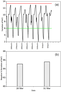Figure 1

We analyzed the $f_oF2$ data of period from 25 March to 2 April 2014. The results are plotted as shown in Figure 1a and Figure 1b. In Figure 1a the upper line represents upper bound and the lower line represents lower bound. As evident from Figure 1b it was found that there is max. 65.14 and 65.64 negative % deviation of $f_oF2$ on 28th and 31st March 2014. The relative negative deviation (% Dev) was from 36.0 to 65.64%.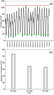Figure 2

Thus 28th and 31st March are the anomalous days prior to earthquake event of 1 April 2014. These days may be considered as precursors. We analyzed the TEC (TECU) data of period from 15 March to 2 April 2014. The results are plotted as shown in Figure 2a and Figure 2b. In Figure 2a the upper line represents upper bound and the lower line represents lower bound. From Figure 2b it was found that there is max. 53.42, 34.80 and 33.50 negative % deviation of TEC on 16th, 22nd, 31st March 2014. The relative negative deviation (% Dev) was from 22.47 to 53.42%. Thus 16th, 22nd, 31st March are the anomalous days prior to earthquake event of 1 April 2014.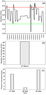Figure 3

We analyzed the $f_oE_s$ data of period from 15 March to 2 April. The results are plotted as shown in Figure 3a and Figure 3b. In Figure 3a the upper line represents upper bound and the lower line represents lower bound. The analysis of $f_oE_s$ data showed that maximum 41.46 negative % deviations of $f_oE_s$ were observed only on 27th March 2014. Also some variations of 1.2–11.6% in upper bound of IQR were observed. The maximum positive deviation was observed on 27th and 29th March 2014. Thus 27th and 29th March are the anomalous days prior to earthquake event.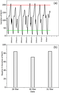Figure 4

The 27th March may be considered as a precursor day. We analyzed the $N_mF2$ data of period from 25 March to 2 April 2014. The results are plotted as shown in Figure 4a and Figure 4b. The values of greatest electron density $N_mF2$ were calculated by formula $N_mF2 = 1.24 \times 10^4(f_oF2)^2$, where $f_oF2$ stands for greatest (critical, penetration) frequency of the ionosphere in MHz. From Figure 4b 84.39 and 84.84 negative % deviation of $N_mF2$ were observed on 28th and 31st March 2014. The relative negative deviation (% Dev) was from 71.26 to 84.84%. Thus 28th and 31st March are the anomalous days prior to earthquake event. The 28th and 31st March may be considered as a precursor day.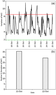Figure 5

In our study ionospheric variations were also examined during the period 18 October to 25 October 2013 prior to ($M=7.1$) Japan earthquake occurred on 25 October 2013 in Japan. We examined the anomalous variation of ionospheric $f_oF2$, $f_oE_s$ and TEC data by analyzing $f_oF2$, $f_oE_s$ and TEC data. The variations in the $Dst$ index were within normal limit values. The $Dst$ index was $Dst > -20$ nT. The value of $Kp$ index was also below 4. This indicates that the space environment and the geomagnetic activity were relatively quiet during the analysis period of second event. The results related to these earthquakes are plotted as shown in Figure 5a to Figure 5b.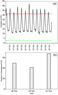Figure 6

We analyzed the $f_oF2$ (MHz) data of period from 17 October to 25 October 2013. The results are plotted as shown in Figure 5a and Figure 5b. In Figure 5a the upper line represents upper bound but the line representing lower bound lie on horizontal $x$ axis The anomalous ionospheric enhancements up to 35.67% and 29.47% from the upper bound of IQR were observed on 21st October and 22nd October 2013. The relative positive deviation (% Dev) was from 25.96–35.67%. Thus 21st and 22nd October are the anomalous days prior to earthquake event. These days may be considered as precursor day. We analyzed the TEC (TECU) data of period from 15 October to 26 October 2013. The results are plotted as shown in Figure 6a and Figure 6b. In Figure 6a the upper line represents upper bound and the lower line represents lower bound. The obtained results show that the TEC parameters were disturbed significantly and positively 33.92% on 21st October. The relative positive deviation (% Dev) was from 5–33.92%. Thus 21st October is the anomalous day prior to earthquake event. This day may be considered as precursor day.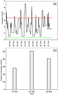Figure 7

We analyzed the $f_oE_s$ (MHz) data of period from 18 October to 27th October 2013.The results are plotted as shown in Figure 7a and Figure 7b. In Figure 7a the upper line represents upper bound and the lower line represents lower bound. The analysis of $f_oE_s$ data showed that 28.5%, 50.9%, and 40.95% positive deviations of $f_oE_s$ were observed on 21st, 22nd and 23rd October 2013. The maximum positive deviation was observed on 22nd October 2013. Thus 21st, 22nd and 23rd October are the anomalous days prior to this earthquake event. These days may be considered as precursor days.

For Chile event we found anomalous variations of $f_oF2$ parameter on 28th March 3 days before and max. variation on 31st March 1 day before earthquake, found anomalous variation of TEC on 16th March (max. variation), 22nd and 31st March 15 days, 9 days, 1 day before earthquake and anomalous variations in $N_mF2$ parameter found on 28th March 3 days before and 31st March (max. variation) 1 day prior to earthquake. For $f_oE_s$ parameter the anomalous variations were observed on 27th March 2014. Thus for Chile event 16th March, 27th March and 31st March are the anomalous days prior to earthquake event. Thus 16th March, 27th March and 31st March may be regarded as precursor day prior to earthquake event. Thus precursors were obtained which was on 15 days, 4 days and 1 day before the Chile earthquake event. For Chile earthquake event the variations in the $Dst$ index and value of $Kp$ index were within normal limit values. This indicates that the space environment and the geomagnetic activity were relatively quiet during the analysis period. Therefore the ionospheres anomaly was probably related to the earthquake event. The results related to Japan earthquake are as shown in Figure 5 to Figure 7. The anomalous variations in $f_oF2$ parameter were observed on 21st October 3 days prior to event. The TEC parameters were found anomalous on 21st October 3 days prior to earthquake. But anomalous variations in $f_oE_s$ observed on 21st October 3 days prior and maximum on 22nd October 2 days prior to earthquake event. Thus 21st and 22nd October are the anomalous days which may be considered as precursor days prior to earthquake event. Thus precursors were obtained on 3 days and 2 days before the Japan earthquake event. The above results of anomalous ionospheric variations seem to be linked with earthquakes.

### 4. Conclusion

For Chile event 16th March, 27th March and 31st March were observed as anomalous days prior to earthquake event. Thus these may be regarded as precursor day prior to earthquake event. Similarly for Japan earthquake event the 21st and 22nd October were observed as anomalous days which may be considered as precursor days prior to earthquake event.

#### Acknowledgment

The authors would like to thank National Geophysical Data Center (NGDC), Space Physics Interactive Data Resource (SPIDR) website at http://spidr.ngdc.noaa.gov/ spidr/ for the $f_oF2$, $f_oE_s$ data support and Ionolab for TEC data support.

### References

Antselevich, M. G. (1971), The influence of Tashkent earthquake on the Earth's magnetic field and the ionosphere, Tashkent Earthquake 26 April 1966, p. 187–188, FAN Publ., Tashkent.

Chuo, Y. J., Y. I. Chen, J. Y. Liu, S. A. Pulinets (2001), Ionospheric $f_oF2$ Variations prior to strong earthquakes in Taiwan area, Adv. Space Res., 27, no. 6–7, p. 1305–1310, doi:10.1016/S0273-1177(01)00209-5.

Chauhan, V., O. P. Singh, U. Pandey, B. Singh, B. R. Arora, G. Rawat, B. M. Pathan, A. K. Sinha, A. K. Sharma, A. V. Patil (2012), A Search for precursors of earthquakes from multi-station ULF observations and TEC measurements in India, Indian J. Radio and Space Sci., 41, p. 543–556.

Dabas, R. S., M. Das Rupesh, Kavita Sharma, K. G. M. Pillai (2007), Ionospheric pre-cursors observed over low latitudes during some of the recent major earthquakes, J. Atm. and Sol.-Terr. Phys., 69, p. 1813–1824, doi:10.1016/j.jastp.2007.09.005.

Datchenko, E. A., V. I. Ulomov, C. P. Chernyshova (1972), Electron density anomalies as the possible precursor of Tashkent earthquake, Dokl. Uzbek. Acad. Sci., 69, no. 12, p. 30–32.

Dobrovolsky, I. R., S. I. Zubko, V. I. Mayachkin (1979), Estimation of the size of earthquake preparation zones, Pure and Appl. Geophys., 117, no. 5, p. 1025–1044, doi:10.1007/BF00876083.

Gokhberg, M. B., V. A. Pilipino, O. A. Pokhotelov (1983), Seismic Precursors in the ionosphere, Izvestiya Earth Phys., 19, p. 762–765.

Liu, J. Y., Y. J. Chuo, S. J. Shan, Y. B. Tsai, S. A. Pulinets, S. B. Yu (2004), Pre-earthquake Ionospheric anomalies monitored by GPS TEC, Ann. Geophys., 22, p. 1585–1593, doi:10.5194/angeo-22-1585-2004.

Plotkin, V. V. (2003), GPS detection of ionospheric perturbation before the 13 February 2001 El Salvador earthquake, Nat. Hazards and Earth Syst. Sci., 3, p. 249–253, doi:10.5194/nhess-3-249-2003.

Pulinets, S. A., K. A. Boyarchuk (2004), Ionospheric Precursors of Earthquakes, 315 pp., Springer, Berlin.

Xu, T., J. Wu, Z. Zhao, Y. Liu, S. He, J. Li, Z. Wu, Y. Hu (2011), Monitoring ionospheric variations before earthquakes using the vertical and oblique sounding network over China, Nat. Hazards Earth Syst. Sci., 11, p. 1083–1089, doi:10.5194/nhess-11-1083-2011.

Received 1 March 2016; accepted 10 March 2016; published 17 March 2016.Citation: Patil Vivek, Roshni Atulkar, P. K. Purohit (2016), Analysis of ionospheric parameters above active seismic regions, Russ. J. Earth Sci., 16, ES1004, doi:10.2205/2016ES000564.

Generated from LaTeX source by ELXpaper, v.1.5 software package.